• 电路以压控放大芯片VCA810为核心，采用基于四阶切比雪夫带通滤波器的模拟电路设计方法。通过EDA电路电路设计软件完成电路板设计与元器件选取，利用LabVIEW虚拟仪器软件与实验室仪器建立连接，实现软硬件协同测试，...
• 要求 LO带通滤波器，在500Hz和Z000Hz处的衰减为3dB，在lOOHz和4000Hz处的最小衰减为4OdB，Rs=RL=600Ω。 　解 ①由于上截止频率和下截止频率之比为4：1，因此可以用宽带设计方法。设计指标首先被分解为单独的低通和...
• 针对多频点蓄电池内阻测试法双低频测试信号滤波问题，设计基于MAX267有源带通滤波器芯片的可选频4阶切比雪夫带通滤波器。通过改变基准输入时钟频率信号，实现带通滤波器通带中心频率的切换。详细介绍了这种前置变频...
• 距离第一篇Butterworth滤波器设计博文之后已经过了一年半，当时说过要把切比雪夫滤波器、椭圆滤波器和贝塞尔滤波器都介绍的，由于各种原因拖到现在，自己挖的坑还是要填的。这里先总体介绍下数字滤波器的设计步骤和...
        距离第一篇Butterworth滤波器设计博文之后已经过了一年半，当时说过要把切比雪夫滤波器、椭圆滤波器和贝塞尔滤波器都介绍的，由于各种原因拖到现在，自己挖的坑还是要填的。这里先总体介绍下数字滤波器的设计步骤和方法。

一、滤波器的分类

按照滤波器的实现方式分为模拟滤波器和数字滤波器；

按照频带分为低通、高通、带通和带阻；

按照实现的元件分为有源滤波器和无源滤波器等；

按照传递函数或者最佳逼近特性分为巴特沃斯滤波器（Butterworth）、贝塞尔滤波器（Bessel）、切比雪夫滤波器（Chebyshev）和椭圆滤波器（Elliptic）等。

二、设计步骤

（1）数字滤波器性能指标；

（2）将数字性能指标转换为模拟指标；

（3）设计模拟低通滤波器或者原型模拟低通滤波器；

（4）原型模拟滤波器频率变换，设计低通、高通、带通和带阻；

（5）冲激不变法或者双线性变化法将模拟滤波器系数、转换为数字滤波器系数、；

（6）设计滤波器的实现结构，由差分方程计算滤波前后信号。

三、设计方法

设计一个滤波器通常会给出如下四个参数：通带截止频率 、阻带截止频率 、通带波纹幅度或者通带衰减率和阻带波纹幅度或者阻带衰减率 ，如下图1所示。

对于数字滤波器设计中使用的几种频率进行说明：

模拟频率 ：每秒经历多少个周期，单位Hz；

采样频率 ：每秒从连续信号中提取并组成离散信号的采样个数，单位Hz。

它们之间的关系如下：

数字滤波器设计常采用间接设计法，即先将给定的数字滤波器技术指标转变为模拟滤波器低通滤波器的技术指标，然后将设计好的低通模拟滤波器转变为所需的数字滤波器，主要原因是模拟滤波器的设计方法比较成熟。从模拟滤波器到数字滤波器的设计方法有冲激响应不变法和双线性变换法等，以双线性变换法应用最广泛。用双线性变换法设计IIR数字滤波器的步骤如图2所示。


展开全文• 有源滤波器精确设计手册pdf,概括介绍了有源滤波器的种类和结构特点。接着分开详细介绍了巴特沃斯和切比雪夫低通滤波器，带通滤波器，带阻滤波器，全通和恒定延时滤波器的特点和实际的设计方法开关电源
• 基于MATLAB的IIR和FIR滤波器设计-实验4 FIR数字滤波器设计.doc IIR：在MATLAB中，可以用下列函数辅助设计IIR数字滤波器：1)利用buttord和cheb1ord可以确定低通原型巴特沃斯和切比雪夫滤波器的阶数和截止频率；2)...matlab
• 基于MATLAB的IIR和FIR滤波器设计-实验5 基于MATLAB的数字滤波器设计.doc IIR：在MATLAB中，可以用下列函数辅助设计IIR数字滤波器：1)利用buttord和cheb1ord可以确定低通原型巴特沃斯和切比雪夫滤波器的阶数和截止...matlab
• 　2.5 m推演型LPF的归一化设计数据及滤波器设计方法 　2.6 m推演型滤波器与定K型滤波器的组合设计 　2.7 利用m推演型改善匹配性的滤波器设计技术 第3章 巴特沃思型低通滤波器的设计——以其通带衰减特性平坦而闻名，...
• 本书作为一本介绍LC滤波器设计和制作方法的实用性图书，内容包括了经典设计方法和现代设计方法，如定K型、m推演型、切比雪夫、巴特沃斯、贝塞尔、逆切比雪夫、椭圆函数型等低通、高通、带通、带阻滤波器以及电容耦合...
• 巴特沃思、切比雪夫模拟低通滤波器通常是设计模拟高通、带通和带阻滤波器的原型,先按给定频率响应巴特沃思、切比雪夫低通Ha(s)逼近,然后由选定Ha(s)实现二端口网络的电路结构和参数值。在此对达林顿T型和П型电路...
• 滤波器作为信号处理的重要组成部分已发展的相当成熟本论文首先介绍了滤波器的滤波原理以及模拟滤波器数字滤波器设计方法重点介绍了模拟滤波器的设计和仿真系统研究了模拟滤波器包括巴特沃斯滤波器切比雪夫滤波器...
• 本书作为一本介绍LC滤波器设计和制作方法的实用性图书，内容包括了经典设计方法和现代设计方法，如定K型、m推演型、巴特沃思型、切比雪夫型、贝塞尔型、高斯型、逆切比雪夫型、椭圆函数型等低通、高通、带通、带阻...
• 本书作为一本介绍LC滤波器设计和制作方法的实用性图书，内容包括了经典设计方法和现代设计方法，如定K型、m推演型、巴特沃思型、切比雪夫型、贝塞尔型、高斯型、逆切比雪夫型、椭圆函数型等低通、高通、带通、带阻...
• 1. 学习双线性变换法及脉冲响应不变法设计IIR数字滤波器的具体设计方法及其原理，利用双线性变换法或脉冲响应不变法设计低通、高通和带通其中一种IIR数字滤波器。 2. 观察双线性变换或脉冲响应不变法设计的滤波器的...
• 巴特沃思、切比雪夫模拟低通滤波器设计方法；利用模拟域频率变换设计模拟高通、带通、带阻滤波器的方法。利用脉冲响应不变法、双线性变换法设计数字滤波器的基本方法；设计巴特沃思、切比雪夫低通、带通、高通、带...
• 为了实现多通道声发射监测仪中对从传感器接收到的声发射信号进行低通三档和高通三档的程控滤波，基于可程控开关电容有源滤波器MAX262，设计出了一种由低通滤波器和高通滤波器级联而成的切比雪夫带通滤波器，并通过...
• 2、波纹3、带宽4、矩形系数5、品质因数8.3 滤波器基本分析方法1、镜像参数法2、网络综合法8.4 低通滤波器原型8.4.1 巴特沃斯滤波器8.4.2 线性相移滤波器（贝塞尔滤波器）8.4.3 切比雪夫滤波器8.5 滤波器设计方法...
这里写目录标题8.1 常见的射频滤波器8.1.1   LC滤波器8.1.2   晶体滤波器8.1.3   陶瓷滤波器8.1.4   声表面波滤波器8.2  滤波器的基本结构滤波器的主要技术指标1、通带插入损耗2、波纹3、带宽4、矩形系数5、品质因数${Q_{L}}$8.3  滤波器基本分析方法1、镜像参数法2、网络综合法8.4 低通滤波器原型8.4.1 巴特沃斯滤波器8.4.2 线性相移滤波器（贝塞尔滤波器）8.4.3 切比雪夫滤波器8.5 滤波器设计方法8.5.1 频率变换低通→低通 频率变换低通→高通 频率变换低通→带通 频率变换低通→带阻 频率变换8.5.2 阻抗变换例题 设计带通滤波器滤波器阶数的确定1、巴特沃斯滤波器设计巴特沃斯低通滤波器2、切比雪夫滤波器设计带通切比雪夫滤波器作业
8.1 常见的射频滤波器
8.1.1   LC滤波器
由电感L和电容C元件构成的滤波器称为LC滤波器
通常可分为一般LC滤波器、谐振回路滤波器和耦合回路LC滤波器
一般LC滤波器可实现低通、高通、带通和带阻等各种功能
谐振回路LC滤波器一般只能实现带通和带阻（或陷波）两种功能
耦合回路LC滤波器通常仅实现带通的功能。
8.1.2   晶体滤波器
晶体谐振器不但可以用作振荡器还可以用来制作滤波器
晶体滤波器具有体积小和重量轻的优点，并且由于晶体的Q值很高，易于实现窄带的带通或带阻滤波器。
晶体滤波器具有中心频率稳定，带宽窄，边沿衰减陡峭的特点
晶体滤波器的相对带宽只有千分之几，在许多情况下限制了其应用。
8.1.3   陶瓷滤波器
压电陶瓷材料经直流高压电场极化后，可以得到类似于石英晶体中的压电效应，其等效电路也和晶体谐振器相同
但陶瓷滤波器的品质因数较晶体小得多（约为几百），但比LC滤波器的品质因数高，且串、并联频率间隔也比较大
因此，陶瓷滤波器的相对带宽较大。高频陶瓷滤波器的工作频率可以从几兆赫至到上百兆赫，并且其相对带宽可从千分之几至10％。
简单的陶瓷滤波器是在单片压电陶瓷上形成双电极或三电极，它们相当于单振荡回路或耦合回路
性能较好的陶瓷滤波器通常是将多个陶瓷谐振器接入梯形网络而构成
它是一种多极点的带通（或带阻）滤波器
单片陶瓷滤波器通常用在放大器射极电路中取代旁路电容
由于陶瓷滤波器的Q值比通常电感元件高，滤波器的通带衰减小，带外衰减大，矩形系数较小。
8.1.4   声表面波滤波器
声表面波滤波器（SAW）器件是一种利用沿弹性固体表面传播机械振动波的器件
在压电固体材料表面产生和传播、且振幅随深入固体材料的深度增加而迅速减小的弹性波称为声表面波（SAW），它具有能量密度高和传播速度慢的特点。
在声表面波滤波器中，叉指换能器一般是均匀的，也可以对指长、指宽或者叉指间隔进行加权，这样就可以得到幅频特性（选择性）更好，或者满足特殊幅频特性要求的滤波器。
8.2  滤波器的基本结构
教材135页 PDF152页实际的滤波器实现形式是根据不同的传递函数对理想特性的逼近，可以分为巴特沃斯、切比雪夫、椭圆函数等基本滤波器，它们的幅度频率响应如下图所示：滤波器的主要技术指标
1、通带插入损耗
理想的滤波器不应对通带内的信号引入损耗，然而实际的电路中总是会引入一定的功率损耗，称为插入损耗
通带插入损耗 = 信号源输入功率${P_{in}}$ / 负载得到的功率${P_{L}}$
$IL(dB) = 10\log \frac{{P_{in}}}{P_{L}} = - 10\log (1 - {\left| {{\Gamma _{in}}} \right|^2})$
$IL(dB) = 10\log \frac{{P_{A}}}{P_{L}}$★(5.1)
${P_{A}}$波源资用功率
${\Gamma _{{\rm{in}}}}$是从信号源向滤波器看去的反射系数
2、波纹
波纹是衡量带内响应平坦度的技术指标
可以用波纹系数定量的分析波纹的起伏大小
定义为带内用分贝表示的相应幅度的最大值 - 相应幅度的最小值
3、带宽
对于带通滤波器不同的衰减量对应于不同的带宽，常用的有
3dB带宽定义为通带内幅度衰减为3dB的上下边带之差
60dB带宽定义为通带内幅度衰减为60dB的上下边带之差
4、矩形系数
矩形系数描述了带通滤波器通带与阻带间过渡带宽的陡峭程度
通常定义为60dB带宽${BW_{60dB}}$ / 3dB带宽${BW_{3dB}}$
$SF = {BW_{60dB}}/{BW_{3dB}}$★(5.3)
5、品质因数${Q_{L}}$
品质因数可以分为空载品质因数Q和有载品质因数${Q_{L}}$
两者由是否接有负载区分
通常空载品质因数 Q定义为在谐振频率下，滤波器上一个周期内平均储能与功率损耗的比：
品质因数${Q_{L}}$与3dB带宽${BW_{3dB}}$间的关系
${Q_{L}}={f_{c}}/{BW_{3dB}}$★(5.7)
${BW_{3dB}} = {f_{c}}/{{{Q_{\rm{L}}}}}$
相对带宽$={BW_{3dB}}/{f_{c}}=1/{Q_{L}}$
8.3  滤波器基本分析方法
射频滤波器分许多种类，各有不同的特点
但在电路中能灵活设计的主要有集总元件的LC滤波器和分布参数的耦合线滤波器
本章主要介绍集总元件参数LC滤波器的设计思想和设计方法。
LC滤波器设计方法主要有两种。
1、镜像参数法
镜像参数法以基本$\Gamma ,T,\Pi$型网络结构为基本单元。规定约束条件${Z_1}{Z_2} = {R^2}$
其中：R为设计常数。
再用ABCD矩阵分析其网络衰耗特性与输入输出阻抗特性
由于ABCD矩阵适于分析网络级联，因此也可扩展到多级网络的分析与设计。
2、网络综合法
网络综合法以归一化低通滤波器的传统函数为基础
首先用多项式逼近法构建满足滤波器衰耗条件的传递函数
再建立传递函数与阻抗函数的关系
用阻抗函数建立梯形网络的实现原型
最后经过频率变换和阻抗变换，获得实际需要的滤波器
我们重点介绍该方法的基本原理及设计方法。8.4 低通滤波器原型
所谓滤波器原型是指归一化低通滤波器模型（截止频率为1，信号源内阻为1），滤波器原型确定了滤波器的特性
然后通过原型滤波器的频率变换，阻抗变换等获得实际需要的滤波器。
8.4.1 巴特沃斯滤波器
巴特沃斯滤波器插入损耗
$IL = 10\log \left( {1 + {a^2}{\Omega ^{2N}}} \right)$★
归一化频率${{\Omega = \omega } \mathord{\left/ {\vphantom {{\Omega = \omega } {{\omega _c}}}} \right.} {{\omega _c}}}$
N为滤波器阶数
a为通带衰耗系数，通常可以令a=1，将a值合并到归一化频率之中去。得
$IL = 10\log \frac{1}{{1 - {{\left| {{\Gamma _{in}}(j\Omega )} \right|}^2}}} = 10\log \left( {1 + {\Omega ^{2N}}} \right)$★
8.4.2 线性相移滤波器（贝塞尔滤波器）
8.4.3 切比雪夫滤波器
切比雪夫滤波器插入损耗
$IL = 10\log \left\{ {1 + {a^2}C_n^2(\Omega )} \right\}$★
8.5 滤波器设计方法
设计流程：
1、根据设计指标确定归一化设计模型及参数
2、进行频率变换，将归一化低通模型变换成实际的模型
如：高通，带通，帯阻等
及将归一化频率变换为实际频率
并调整其元件值。
3、进行阻抗变换，将归一化阻抗变换成为系统需要的阻抗、即特征阻抗
同时进一步调整元件值。
8.5.1 频率变换
将归一化频率变换成实际频率，同时变换模型。
低通→低通 频率变换
低通→低通频率变换
$\Omega = \left( {\frac{\omega }{{{\omega _C}}}} \right)$★
${\omega _C}$实际截止频率，对应于归一化截止频率1
元件值变换：（L、C为归一化元件值）
${Z_L} = j\Omega L = j\frac{\omega }{{{\omega _c}}}L = j\omega L'{\rm{ }} \Rightarrow {\rm{ }}L' = \frac{L}{{{\omega _c}}}$★
${Y_C} = j\Omega C = j\frac{\omega }{{{\omega _c}}}C = j\omega C'{\rm{ }} \Rightarrow {\rm{ }}C' = \frac{C}{{{\omega _c}}}$★
低通→高通 频率变换
低通→高通频率变换
$\Omega = \frac{{ - {\omega _c}}}{\omega }$★
元件值变换：
电感→电容
$Z = j\Omega L = - j\frac{{{\omega _c}}}{\omega }L = \frac{1}{{j\omega C'}}{\rm{ }} \Rightarrow C' = \frac{1}{{{\omega _c}L}}$★
电容→电感
$Y = j\Omega C = - j\frac{{{\omega _c}}}{\omega }C = \frac{1}{{j\omega L'}}{\rm{ }} \Rightarrow L' = \frac{1}{{{\omega _c}C}}$★
注意：虽然器件的性质改变，当在网络中的位置不变。
低通→带通 频率变换
一般带通滤波器设计是

确定归一化滤波器，将带通滤波器指标变换为归一化低通滤波器指标
通过频率变换和阻抗变换获得实用滤波器

低通→带通频率变换
$\Omega = \frac{{{\omega _0}}}{{{\omega _U} - {\omega _L}}}\left( {\frac{\omega }{{{\omega _0}}} - \frac{{{\omega _0}}}{\omega }} \right)$，${\omega _U}{\omega _L} = \omega _0^2$★
$\Omega = \frac{{{f_0}}}{{{f_U} - {f_L}}}\left( {\frac{f}{{{f_0}}} - \frac{{{f_0}}}{f}} \right)$，${f_U}{f_L} = f_0^2$★
$\omega {\rm{ = }}{\omega _U} \Rightarrow \Omega=1$
$\omega {\rm{ = }}{\omega _L} \Rightarrow \Omega=1$
$\omega {\rm{ = }}{\omega _0} \Rightarrow \Omega=0$
低通→带通变换 原型电路的电感→电感电容串联
$Z = j\Omega L = j\frac{{{\omega _0}}}{{{\omega _U} - {\omega _L}}}\left( {\frac{\omega }{{{\omega _0}}} - \frac{{{\omega _0}}}{\omega }} \right)L = j\frac{{\omega L}}{{{\omega _U} - {\omega _L}}} + \frac{1}{{j\omega \left( {\frac{{{\omega _U} - {\omega _L}}}{{\omega _0^2}}} \right)\frac{1}{L}}}\\ = j\frac{{\omega L}}{{{\omega _U} - {\omega _L}}} + \frac{1}{{j\omega \left( {\frac{{{\omega _U} - {\omega _L}}}{{\omega _0^2}}} \right)\frac{1}{L}}} = j\omega {{L'}_s} + \frac{1}{{j\omega {{C'}_s}}}$
${{L'}_s} = \frac{L}{{{\omega _U} - {\omega _L}}}$★
${{C'}_s} = \frac{{{\omega _U} - {\omega _L}}}{{\omega _0^2L}} = \frac{1}{{\omega _0^2{{L'}_s}}}$★
$\omega _0^2 = \frac{1}{{{{L'}_s}{{C'}_s}}}$★
低通→带通变换 原型电路的电容→电感电容并联
$Y = j\Omega C = j\frac{{\omega C}}{{{\omega _U} - {\omega _L}}} + \frac{1}{{j\omega \left( {\frac{{{\omega _U} - {\omega _L}}}{{\omega _0^2}}} \right)\frac{1}{C}}} = j\omega {{C'}_p} + \frac{1}{{j\omega {{L'}_p}}}$
${{C'}_p} = \frac{C}{{{\omega _U} - {\omega _L}}}$★
${{L'}_p} = \frac{{{\omega _U} - {\omega _L}}}{{\omega _0^2C}} = \frac{1}{{\omega _0^2{{C'}_p}}}$★
$\omega _0^2 = \frac{1}{{{{L'}_p}{{C'}_p}}}$★
低通→带阻 频率变换
低通→带阻频率变换
$\Omega = {\left\{ {\frac{{ - {\omega _0}}}{{{\omega _U} - {\omega _L}}}\left( {\frac{\omega }{{{\omega _0}}} - \frac{{{\omega _0}}}{\omega }} \right)} \right\}^{ - 1}}$★
${\omega _U}{\omega _L} = \omega _0^2$★
低通→带阻变换 原型电路的电感→电感电容并联
$Z = j\Omega L = jL{\left\{ {\frac{{ - {\omega _0}}}{{{\omega _U} - {\omega _L}}}\left( {\frac{\omega }{{{\omega _0}}} - \frac{{{\omega _0}}}{\omega }} \right)} \right\}^{ - 1}} = {\left\{ {\frac{1}{{jL}}} \right\}^{ - 1}}{\left\{ {\left( {\frac{{ - \omega }}{{{\omega _U} - {\omega _L}}}} \right) + {{\left( {\omega \frac{{{\omega _U} - {\omega _L}}}{{\omega _0^2}}} \right)}^{ - 1}}} \right\}^{ - 1}}\\ = {\left\{ {\frac{{j\omega }}{{\left( {{\omega _U} - {\omega _L}} \right)L}} + \frac{{\omega _0^2}}{{jL\omega \left( {{\omega _U} - {\omega _L}} \right)}}} \right\}^{ - 1}} = {\left\{ {j\omega {{C'}_p} + \frac{1}{{j\omega {{L'}_p}}}} \right\}^{ - 1}}$
${{C'}_p} = \frac{1}{{\left( {{\omega _U} - {\omega _L}} \right)L}}$★
${{L'}_p} = \frac{{{\omega _U} - {\omega _L}}}{{\omega _c^2}}L$★
低通→带阻变换 原型电路的电容→电感电容串联
$Y = j\Omega C = {\left\{ {\frac{{j\omega }}{{\left( {{\omega _U} - {\omega _L}} \right)C}} + \frac{{\omega _0^2}}{{jC\omega \left( {{\omega _U} - {\omega _L}} \right)}}} \right\}^{ - 1}} = {\left\{ {j\omega {{L'}_s} + \frac{1}{{j\omega {{C'}_s}}}} \right\}^{ - 1}}$
${{L'}_s} = \frac{1}{{\left( {{\omega _U} - {\omega _L}} \right)C}}$★
${{C'}_s} = \frac{{{\omega _U} - {\omega _L}}}{{\omega _0^2}}C$★
8.5.2 阻抗变换
阻抗变换
特征阻抗$R_G$★
对串联电感支路
$j\Omega {L'_s} = j{R_G}{a_s}\Omega$
${L'_s} = {R_G}{a_s} = {R_G}{L_s}$★
${a_n}:n = 1,3,5,7.....$
对并联电容支路
$j\Omega {C'_p} = j\frac{{{a_p}}}{{{R_G}}}\Omega$
${C'_p} = {{{a_p}}}/{{{R_G}}} = {{{C_p}}}/{{{R_G}}}$★
${a_n}:n = 2,4,6,8.....$
阻抗变换的另一种解释
传递函数值是无量纲比例值，这个值由网络拓扑结构和元件比例值决定，而与元件的绝对值无关
网络中所有元件的阻抗值等比例变化，传递函数不变
这样阻抗变换可用下列公式解释
$Z' = KZ$，$Y' = Y/K$
从归一化电路$R_S=R_L=1$，变化到${R'_S} = R'_L = {R_G}$，有$K=R_G$
$R'_S=R_G R_S$★
$R'_L=R_G R_L$★

★★

★★

$Z = j\omega L$
$Z' = KZ = j\omega {R_G}L = j\omega L'$
$L' = {R_G}L$

$Z = \frac{1}{{j\omega C}}$
$Z' = KZ = \frac{{{R_G}}}{{j\omega C}} = \frac{1}{{j\omega C'}}$
$C' = \frac{C}{{{R_G}}}$

例题 设计带通滤波器归一化低通滤波器原型电路如图所示
将其变换为特征阻抗为50欧，上下截止频率分别在1.01MHz和0.99MHz的带通滤波器
特征阻抗$R_G=50Ω$★
上截止频率${\omega _{\rm{U}}} = 2\pi \times 1.01 \times {10^6} \approx 6.346 \times {10^6}\left( {rad/s} \right)$
下截止频率${\omega _L} \approx 6.220Hz \times {10^6}\left( {rad/s} \right)$
${\omega _{\rm{U}}} - {\omega _L} \approx 0.126 \times {10^6}\left( {rad/s} \right)$
$\omega _0^2 = 3.947 \times {10^{13}}$
$\omega _0 = 6.283 \times {10^6}Hz$
低通→带通变换电感电容串联 阻抗变换
${{L'}_s} = \frac{{{R_G}L}}{{{\omega _U} - {\omega _L}}}{\rm{ = }}\frac{{50 \times \sqrt 2 }}{{0.126 \times {{10}^6}}} \approx {\rm{5}}{\rm{.61}} \times {10^{ - 4}}{\rm{H}} \approx 560\mu H\\ {{C'}_s} = \frac{{{\omega _U} - {\omega _L}}}{{\omega _0^2{R_G}L}} = \frac{{0.126 \times {{10}^6}}}{{3.947 \times {{10}^{13}} \times 50 \times \sqrt 2 }} \approx 4.51 \times {10^{ - 11}} \approx 45pF$
低通→带通变换电感电容并联 阻抗变换
${{L'}_p} = \frac{{{R_G}\left( {{\omega _U} - {\omega _L}} \right)}}{{\omega _0^2C}} = \frac{{50 \times 0.126 \times {{10}^6}}}{{3.947 \times {{10}^{13}} \times \sqrt 2 }} \approx 1.13 \times {10^{ - 7}} \approx 110nH\\ {{C'}_p} = \frac{C}{{{R_G}\left( {{\omega _U} - {\omega _L}} \right)}}{\rm{ = }}\frac{{\sqrt 2 }}{{50 \times 0.126 \times {{10}^6}}} \approx {\rm{2}}{\rm{.24}} \times {10^{ - 7}}{\rm{F}} \approx 0.22\mu F$
阻抗变换
$R'_S=R_G R_S$★
$R'_L=R_G R_L$★
实际带通电路滤波器阶数的确定
1、巴特沃斯滤波器
一般滤波器的衰耗特性有两个要求
通带波动<指标值，低通型表示为
$IL \le I{L_{low}},\left| \omega \right| \le {\omega _L}$
阻带衰耗>指标值，低通型表示为
$IL \ge I{L_{high}},\left| \omega \right| \ge {\omega _H}$
由指标值根据衰耗公式确定滤波器的阶数，然后生成所需滤波器。
设计巴特沃斯低通滤波器
例：根据下列指标设计阻抗为50欧的巴特沃斯低通滤波器
通带衰耗≤2dB $\left| f \right| \le 3400Hz$
阻带衰耗≥10dB $\left| f \right| \ge 4200Hz$
通带边缘频率${\omega _L} = 2\pi \times 3400rad/s$
阻带下限频率${\omega _H} = 2\pi \times 4200rad/s$
巴特沃斯滤波器插入损耗
$IL = 10\log \left( {1 + {a^2}{\Omega ^{2N}}} \right) = 10\log \left( {1 + {a^2}{{\left( {\frac{\omega }{{{\omega _L}}}} \right)}^{2N}}} \right)$
通带衰耗≤$I{L_{low}}= 2dB$
$\left| \omega \right| \le {\omega _L}$
$10\log \left( {1 + {a^2}} \right) \le I{L_{low}}$
${a^2} \le \left( {{{10}^{IL_{low}/10}} - 1} \right) = \left( {{{10}^{0.2}} - 1} \right) \approx 0.585$
$a \le 0.765$
阻带衰耗≥$IL_{high} = 10dB$
$10\log \left( {1 + {a^2}{{\left( {\frac{{{\omega _H}}}{{{\omega _L}}}} \right)}^{2N}}} \right) \ge IL_{high} = 10dB$
${\left( {\frac{{{\omega _H}}}{{{\omega _L}}}} \right)^{2N}} \ge \frac{{{{10}^{IL_{high}/10}} - 1}}{{{a^2}}}$
两边取对数，log的底数取什么都可以
$N \ge \frac{{\log ({{\sqrt {{{10}^{IL_{high}/10}} - 1} }}/{{{a^{}}}})}}{{\log ({{{\omega _H}}}/{{{\omega _L}}})}} = \frac{{\log \frac{{\sqrt {{{10}^1} - 1} }}{{0.765}}}}{{\log \frac{{4200}}{{3400}}}} \approx 6.46$
取整数N=7
巴特沃斯滤波器插入损耗
$IL = 10\log \left( {1 + {a^2}{{\left( {\frac{\omega }{{{\omega _L}}}} \right)}^{2N}}} \right) = 10\log \left( {1 + {\Omega ^{2N}}} \right):\Omega = \left( {\frac{\omega }{{{{{\omega _L}} \mathord{\left/ {\vphantom {{{\omega _L}} {\sqrt[N]{a}}}} \right.} {\sqrt[N]{a}}}}}} \right)$
低通→低通频率变换
$\Omega = \frac{\omega }{{{\omega _c}}}$
${\omega _c} = {{{\omega _L}}}/{{\sqrt[N]{a}}} = {{2\pi \times 3400}}/{{\sqrt{{0.765}}}} \approx 22197rad/s$
题就是查表确定归一化元件值
然后通过阻抗变换和频率变换得元件值
设计可以用阻抗设计（即表中第一项为串联电感）
或者用导纳设计（即表中第一项为并联电容）
假设采用阻抗设计	阻抗变换
${L_1} = {g_1} = {L_7} = 0.445H$
${{L'}_{1,7}} = \frac{{{R_G}}}{{{\omega _c}}}L = \frac{{50}}{{22197}} \times 0.445 \approx {10^{ - 3}}H = 1mH$
${C_2} = {g_2} = {C_6} = 1.247F$
${{C'}_{2,6}} = \frac{1}{{{R_G}{\omega _c}}}C = \frac{{1.247}}{{50 \times 22197}} \approx 1.1 \times {10^{ - 6}}F = 1.1\mu F$
${L_3} = {g_3} = {L_5} = 1.8019H$
${{L'}_{3.5}} = \frac{{{R_G}}}{{{\omega _c}}}L = \frac{{50}}{{22197}} \times 1.8019 \approx 4.1 \times {10^{ - 3}}H = 4.1mH$
${C_4} = {g_4} = 2F$
${{C'}_{2,6}} = \frac{1}{{{R_G}{\omega _c}}}C = \frac{2}{{50 \times 22197}} \approx 1.8 \times {10^{ - 6}}F = 1.8\mu F$
2、切比雪夫滤波器
切比雪夫滤波器插入损耗
$IL = 10\log \left\{ {1 + {a^2}C_n^2(\Omega )} \right\}$★
${C_N}(\Omega ) = \left\{ \begin{array}{l} \cos \left[ {N \times {{\cos }^{ - 1}}\left( \Omega \right)} \right]{\rm{ }}\left| \Omega \right| \le 1\\ \cos h\left[ {N \times {{\cosh }^{ - 1}}\left( \Omega \right)} \right]{\rm{ }}\Omega > 1\\ {\left( { - 1} \right)^N}\cos h\left[ {N \times {{\cosh }^{ - 1}}\left( { - \Omega } \right)} \right]{\rm{ }}\Omega < - 1 \end{array} \right.$
一般滤波器的衰耗特性有两个要求
通带波动<指标值，低通型表示为
$IL \le I{L_{low}}$，$\left| \omega \right| \le {\omega _c}$
阻带衰耗>指标值，低通型表示为
$IL \ge I{L_{high}}$，$\left| \omega \right| \ge {\omega _p}$
由指标值根据衰耗公式确定切比雪夫滤波器的阶数，然后生成所需滤波器。
$\left| {\cos \left[ {N \times {{\cos }^{ - 1}}\left( \Omega \right)} \right]} \right| \le 1$
$10\log (1 + {a^2}) \le I{L_{low}}$
$a \le \sqrt {{{10}^{IL_{high}/10}} - 1}$
$I{L_{high}} \le 10\log \left( {1 + {a^2}{{\cosh }^2}\left[ {N \times {{\cosh }^{ - 1}}\left( {\frac{{{\omega _p}}}{{{\omega _c}}}} \right)} \right]} \right)$
$N \ge {{{{\cosh }^{ - 1}}\left( {{{\sqrt {{{10}^{IL_{high}/10}} - 1} }}/{a}} \right)}}/{{{{\cosh }^{ - 1}}\left( {{{{\omega _p}}}/{{{\omega _c}}}} \right)}}$★
设计带通切比雪夫滤波器
设计满足下列指标的切比雪夫滤波器
标称阻抗=50Ω
通带中心频率=70MHz
通带波动≤3dB $\left( {f \ge 69MHz{\rm{ or }}f \le 71MHz} \right)$
阻带衰耗≥40dB $\left( {f \le 65MHz{\rm{ or }}f \ge 75MHz} \right)$
将技术指标转换为归一化低通滤波器的指标
${\omega _L} = 2\pi \times 69 \times {10^6}rad/s$
${\omega _U} = 2\pi \times 71 \times {10^6}rad/s$
${\omega _0} = 2\pi \times \sqrt {69 \times 71} \times {10^6}rad/s$
频率变换
$\frac{{{\omega _0}}}{{{\omega _U} - {\omega _L}}} = \frac{{{f_0}}}{{{f_U} - {f_L}}} = \frac{{\sqrt {69 \times 71} }}{{71 - 69}}$
低通→带通频率变换
$\Omega = \frac{{{\omega _0}}}{{{\omega _U} - {\omega _L}}}\left( {\frac{\omega }{{{\omega _0}}} - \frac{{{\omega _0}}}{\omega }} \right)$，${\omega _U}{\omega _L} = \omega _0^2$★
$\Omega = \frac{{{f_0}}}{{{f_U} - {f_L}}}\left( {\frac{f}{{{f_0}}} - \frac{{{f_0}}}{f}} \right)$，${f_U}{f_L} = f_0^2$★
将上下两个阻带边界频率带入转换公式计算，得
${\Omega _1} = \frac{{{f_0}}}{{{f_U} - {f_L}}}\left( {\frac{{{f_1}}}{{{f_0}}} - \frac{{{f_0}}}{{{f_1}}}} \right){\rm{ }} = \frac{{\sqrt {69 \times 71} }}{{71 - 69}}\left( {\frac{{66}}{{\sqrt {69 \times 71} }} - \frac{{\sqrt {69 \times 71} }}{{66}}} \right) \approx - 4.1$
${\Omega _2} = \frac{{{f_0}}}{{{f_U} - {f_L}}}\left( {\frac{{{f_2}}}{{{f_0}}} - \frac{{{f_0}}}{{{f_2}}}} \right){\rm{ }} = \frac{{\sqrt {69 \times 71} }}{{71 - 69}}\left( {\frac{{74}}{{\sqrt {69 \times 71} }} - \frac{{\sqrt {69 \times 71} }}{{74}}} \right) \approx 3.9$
因为$\left| {{\Omega _2}} \right| < \left| {{\Omega _1}} \right|$
所以用$\Omega _2$作为阻带指标更为严格。
归一化指标为
$IL \le 3dB,\left| \Omega \right| \le 1$
$IL \ge 40dB,\left| \Omega \right| \ge 3.9$
$a \le \sqrt {{{10}^{IL_{low}/10}} - 1} \approx 1$
切比雪夫滤波器的阶数
$N \ge {{{{\cosh }^{ - 1}}\left( {{{\sqrt {{{10}^{IL_{high}/10}} - 1} }}/{a}} \right)}}/{{{{\cosh }^{ - 1}}\left( {{{{\omega _p}}}/{{{\omega _c}}}} \right)}}\\ = {{{{\cosh }^{ - 1}}\left( {{{\sqrt {{{10}^{IL_{high}/10}} - 1} }}/{a}} \right)}}/{{{{\cosh }^{ - 1}}\left( {{{{\Omega _2}}}/{1}} \right)}} = {{{{\cosh }^{ - 1}}\left( {\sqrt {{{10}^{40/10}} - 1} } /1\right)}}/{{{{\cosh }^{ - 1}}\left( {3.9} \right)}} \approx 2.6$
取N=3
查表得${g_1} = {g_3} = 3.3487$，${g_2} = 0.7117$，${{\rm{g}}_{\rm{4}}} = 1$
g4=1，意味着远端阻抗和负载端阻抗一致
低通→带通变换电感电容串联 阻抗变换
$L = {g_1}{\rm{ }}{{L'}_{s1,3}} = \frac{{{R_G}{g_1}}}{{{\omega _U} - {\omega _L}}}{\rm{ = }}\frac{{{\rm{50}} \times {\rm{3}}{\rm{.3487}}}}{{2\pi \left( {71 - 69} \right) \times {{10}^6}}} \approx {\rm{1}}{\rm{.3}} \times {10^{ - 5}} \approx {\rm{13}}\mu {\rm{H }}$
${{C'}_{s1,3}} = \frac{{{\omega _U} - {\omega _L}}}{{\omega _0^2{R_G}{g_1}}} = \frac{1}{{\omega _0^2{{L'}_s}}} = \frac{1}{{\left[ {{{\left( {2\pi } \right)}^2} \times 69 \times 71 \times {{10}^{12}}} \right] \times {\rm{1}}{\rm{.3}} \times {{10}^{ - 5}}}} \approx 0.4pF$
低通→带通变换电感电容并联 阻抗变换
$C = {g_2}{\rm{ }}{{C'}_p} = \frac{{{g_2}}}{{{R_G}\left( {{\omega _U} - {\omega _L}} \right)}}{\rm{ = }}\frac{{0.7117}}{{50 \times 2\pi \left( {71 - 69} \right) \times {{10}^6}}} \approx 1.1 \times {10^{ - 9}} \approx 1.1nF$
${{L'}_p} = \frac{{{\omega _U} - {\omega _L}}}{{\omega _0^2C}} = \frac{1}{{\omega _0^2{{C'}_p}}} = \frac{1}{{\left[ {{{\left( {2\pi } \right)}^2} \times 69 \times 71 \times {{10}^{12}}} \right] \times 1.1 \times {{10}^{ - 9}}}} \approx 4.6 \times {10^{ - 9}} \approx 4.6nH$
作业
5.9, 5.10, 5.15, 5.16


展开全文• 低通、高通、带通设计方法都要会。窗函数法、最佳逼近法也要会。窗函数比较好记，为什么呢？因为都是以名人命名的。什么汉明窗啊，切比雪夫窗(想想概率论里面是不是也接触到了这个名字？)啊等等，还涉及好多科学家...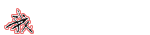上堂课只是简单涉及到了滤波器的基础知识，本堂课的仿真可就要围绕这个滤波器来了。要让大家都能成为滤波器的设计师。低通、高通、带通的设计方法都要会。窗函数法、最佳逼近法也要会。窗函数比较好记，为什么呢？因为都是以名人命名的。什么汉明窗啊，切比雪夫窗(想想概率论里面是不是也接触到了这个名字？)啊等等，还涉及好多科学家的名字。切比雪夫滤波器，又名“车比雪夫滤波器”。这个滤波器在通带或阻带上频率响应幅度是等波纹波动。切比雪夫滤波器来自切比雪夫分布，以“切比雪夫”命名，是用以纪念俄罗斯数学家巴夫尼提·列波维其·切比雪夫。等波纹的特性非常有意思，画出滤波器的频率响应图就能理解了。注明：本章知识对课程《数字信号处理》的消化有很强的促进作用，尤其是对该课程第五章和第六章的知识。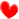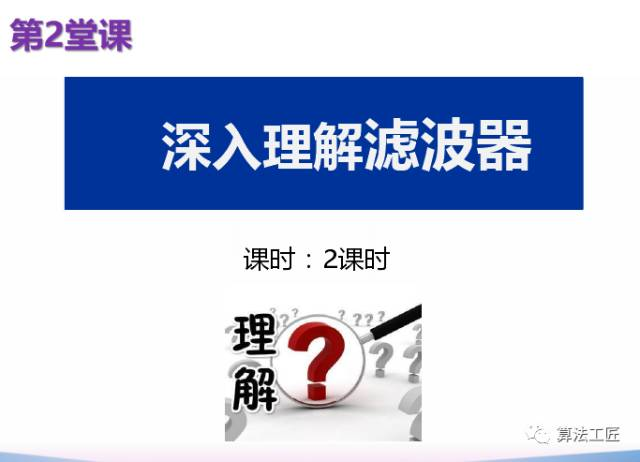看个人的基础，基础好的学生不需要两课时就能掌握滤波器的仿真内容！！！什么是FIR？什么是IIR？需要大家自己回顾复习！温故而知新！！！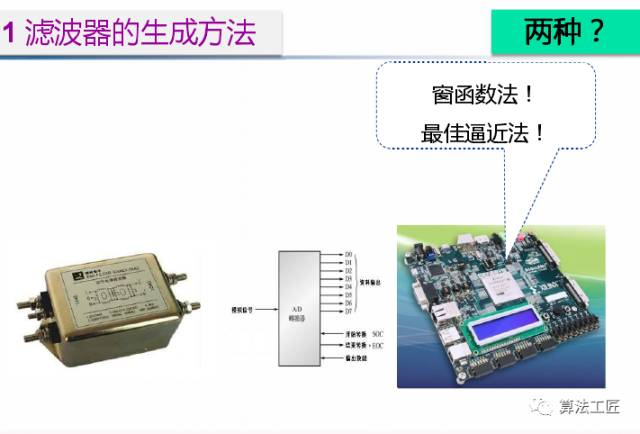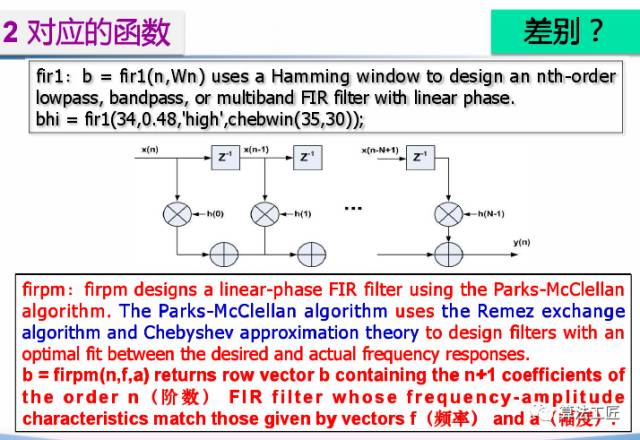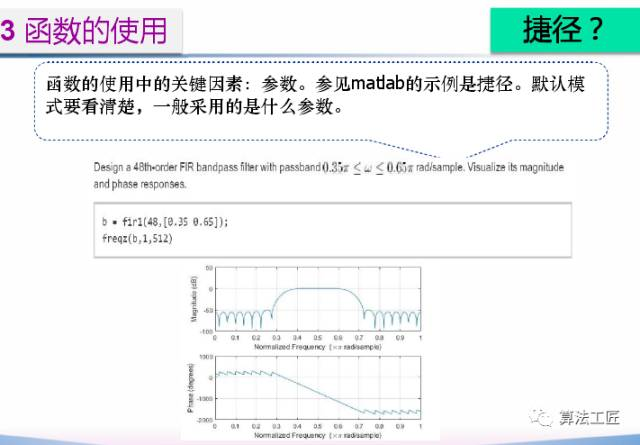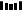本课程侧重仿真，因此同学们一定要能够读懂仿真程序中出现的错误提示，虽然是英文的，但不要成为自己不去读的理由。一定要培养读懂英文资料的习惯！电子通信领域工程师的必由之路！不怕底子差，就怕不想学！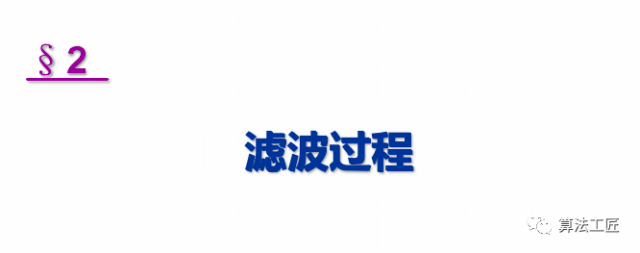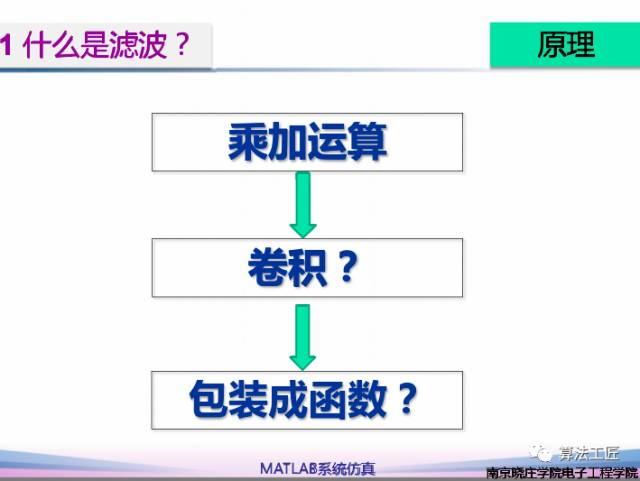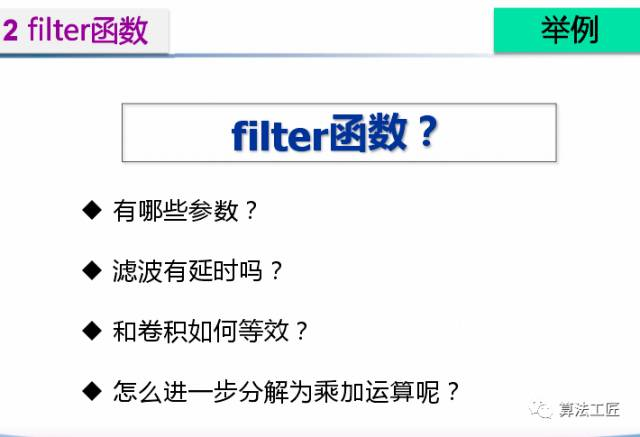重点知识：能否用卷积函数来代替呢？怎么等效使用？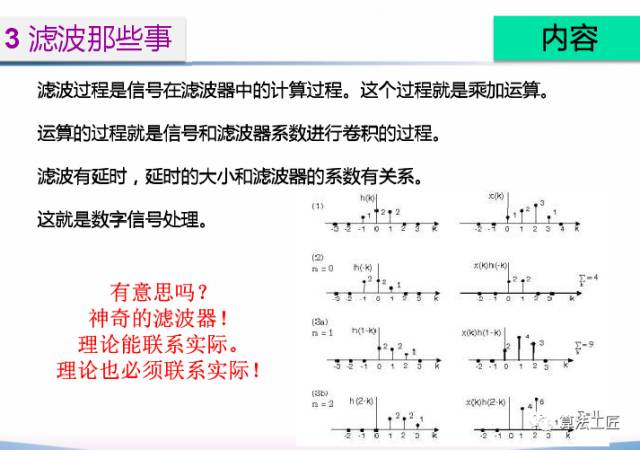未完，待续！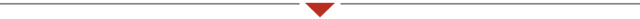学生感言我的一位学生打工了两个月了，偶尔和他聊了工作的事情。没想到他成长挺快。比如两天内发生的事情就让他想到这么多。1.上司面前永远不要解释。2.男人要大度吃点亏不代表就是不好的。3.公司的人际关系是非常重要的，上头说你错，哪怕你是对的，哪怕别人都知道上司是带个人情绪在处理事情，一个人都不帮你说话，你这个职位就危险了。4.公司要的不是有脾气的人，而是有能力又会处理关系的人。5.上司说你错的话可能是不对的，但是他后面说的应该怎么做肯定是对的。6.工作一定要认真仔细，公司的规定很严格，如果你的上司跟你关系比较好，你又确实犯了错，老板讨厌的不会是犯错的员工，而是玩忽职守的管理者。点评：他上了几天班后，确实懂事多了。哎，很难想象又瘦又矮的小伙被训的时候是一幅什么场景？应该是动图。和社会相比，大学依旧是温室。但似乎他做事的风格没什么改变，还是比较拖沓。好习惯很难养成，坏习惯也很难改。2020年回望2017年写的内容，感觉自己的教育之路应该是失败的，所以以后专注于传播知识！！！最后依旧是来段小视频放松一下。学生拍的！修订记录20170127  完成初稿；20170329  修订内容；20170517  修订内容；20201005  修订内容；凡事小小说：出租屋的生活！凡事小小说：衣服没变，人变了！电气信息类专业课程之matlab系统仿真 第一章 信号和滤波器通信原理与matlab仿真v2 第十二章 QPSK相干解调仿真(12)


展开全文matlab 滤波器 matlab滤波 matlab滤波器
• 滤波器学习笔记1 设计一款滤波器首先需要利用广义切比雪夫多项式得到S参数曲线,然后将S曲线转化成为y矩阵。...这里给出了广义切比雪夫滤波器低通原型S曲线的设计方法。 符号说明 为了简化计算,在ω\
滤波器学习笔记1

设计一款滤波器首先需要利用广义切比雪夫多项式得到S参数曲线,然后将S曲线转化成为y矩阵。之后采用提取留数的方法综合出耦合矩阵的主对角线,然后用Givens变换简化耦合矩阵,最终得到滤波器的各种矩阵形式。

广义切比雪夫多项式的综合
要设计一款滤波器首先要得到满足指标要求的S曲线。高通,低通,带通,带阻等各种形式的S曲线要从低通原型变换而来。因此设计滤波器低通原型的S曲线是设计滤波器的第一步。
这里给出了广义切比雪夫滤波器低通原型S曲线的设计方法。

符号说明

为了简化计算,在$\omega$平面上展开讨论。这样,关于$\omega$的多项式的系数都变成了实系数。基于$s=j\omega+\sigma$作变换。变换后会对以s参数为自变量函数产生一些影响。这里不进行讨论。直接使用变换后函数展开讨论。
$S_{11}(\omega)$和$S_{21}(\omega)$代表滤波器的反射系数和传输系数。
滤波器的传输系数和反射系数可以表示为两个多项式的比值,其数学语言描述如下: $S_{11}(\omega) = \frac{F(\omega)/\epsilon_R}{E(\omega)}$ $S_{21}(\omega)=\frac{P(\omega)/\epsilon}{E(\omega)}$
上式中$\epsilon_R$式用来归一化$F(\omega)$和$E(\omega)$的常数,使得他们的最高次项的系数为1,如果最高次项已经等于1则 $\epsilon_R$等于1。
而 $\epsilon=\frac{1}{\sqrt{10^{RL/10}-1}}|\frac{P(\omega)}{F(\omega)/\epsilon_R}|_ {\omega= \pm 1}$ 其中$RL$的值为滤波器在$w=\pm1$ 时的回波损耗。
符号$C_N(\omega)$为N阶滤波器函数,其广义切比雪夫形式为:
$C_N(\omega)=cosh[\sum^N_{n=1}arcosh(x_n(\omega))]$
其中$x_n(\omega)=\frac{1-\omega\omega_n}{\omega-\omega_n}$ ,$\omega_n$为滤波器低通原型的第n个传输零点。

理论推导
为了得到S曲线,首先要得到多项式$F(\omega),P(\omega),E(\omega)$。由于存在能量守恒(无耗)的约束条件,实际只需要得到$F(\omega),P(\omega)$就可以确定$E(\omega)$的值。传输零点是设计者自己确定的,因而下面的推导都基于传输零点已知的条件。也就是$x_n(\omega)$已知。
首先假设$y=arcosh(x_n(\omega))$于是有 $x_n(\omega)=cosh(y)$,然后有$1-x_n(\omega)^2 = sinh(y)^2$推得$arcosh(x_n(\omega))=ln(a_n+b_n)$其中$a_n=x_n(\omega)$,$b_n=\sqrt{x_n(\omega)^2-1}$
将上述结果带入到$C_N(\omega)$中有
$C_N(\omega)=\frac{1}{2}[\prod_{n=1}^{N}(a_n+b_n)+\prod_{n=1}^{N}(a_n-b_n)]$
将$x_n(\omega)$的表达式代入$a_n,b_n$中,最后$C_N(\omega)$的表达式就可以写为
$C_N(\omega)=\frac{1}{2}[\frac{\prod^{N}_ {n=1}(c_n+d_n) +\prod^{N}_ {n=1}(c_n-d_n)}{\prod_{n=1}^{N}(1-\omega/\omega_n)}]$
其中$c_n=(\omega-\frac{1}{\omega_n})$,$d_n=\omega^\prime\sqrt{1-\frac{1}{\omega_n^2}}(\omega^\prime=\sqrt{\omega^2-1})$
可以看出,式中分子带$\omega^\prime$的项可以对消,而最后计算出的分子为两个相等的分别来自分子初始式子两个子项的和。因而计算分子中第一项不带$\omega^\prime$的项的和就可以计算出整个分子的值。
又由于存在$C_N(\omega)=\frac{F(\omega)}{P(\omega)}$,所以$C_N(\omega)$的分子多项式的零点与$F(\omega)$相同。求出分子多项式的表达式也就求出了$F(\omega)$
分母多项式$P(\omega)$完全是由传输零点确定的,按照公式计算即可。但值得注意的是在转换回s平面后,如果 $N-n_{fz}$ ( $n_{fz}$ 为传输零点的个数)为偶数,需要在$P(s)$前乘上虚数j。
在得到$P(\omega)$和$F(\omega)$后就可以求$E(\omega)$了,根据正交归一化条件可以得到一个求解$E(\omega)$的流程。这里不做推导,直接给出流程。
构造多项式 $P(\omega)/\epsilon-jF(\omega)/\epsilon_R$ 求出它的零点,然后求下半平面零点的共轭(为了满足赫尔维茨条件)。再利用上半平面零点(包含求共轭得到的零点)将 $E(\omega)$ 重构出来。
这样得到 $F(\omega),P(\omega),E(\omega)$ 以后,通过简单的计算就得到了S参数。

程序编写

给出了一个简单的程序完成上述过程，选定滤波器阶数为4阶，两个有限传输零点为1.3217和1.8，回波损耗为22dB。
程序主要应用了sympy的符号计算方法，并且生成了低通原型的s11和s21曲线图。

import sympy
import numpy as np
from fractions import Fraction
import matplotlib as mt
import math
# Press the green button in the gutter to run the script.
if __name__ == '__main__':
w1 = 1.3217
w2 = 1.8
wla =  np.array([w1,w2,np.inf,np.inf])
wl = np.array([w2,np.inf,np.inf])
order = 4
w = sympy.symbols("w")
wp = sympy.symbols("wp")
f12 = Fraction(1,2)#用分数sympy可以自己花间
wp = (w**2-1)**f12
U = (w-1/w1)
V = wp*(1-1/w1**2)**f12
for wi in wl:#迭代产生F(w)
Ut = w*U - U/wi + wp*(1-1/wi**2)**f12*V
V = w*V - V/wi + wp*(1-1/wi**2)**f12*U
U = Ut
F = sympy.expand(U)
F = F/F.coeff(w**4)
P = 1
for wi in wla:#迭代产生P(w)
P = P*(1-w/wi)
P = sympy.expand(P)
P = P/P.coeff(w**2)
RL = 22#回波损耗22dB
#计算epsilon
fw1 = F.evalf(subs = {w:1})
pw1 = P.evalf(subs = {w:1})
epsilon = 1/(10**(RL/10)-1)**0.5*abs(pw1/fw1)
coff1 = np.array([P.coeff(w**4),P.coeff(w**3),P.coeff(w**2),P.coeff(w),P.evalf(subs= {w:0})])
coff2 = np.array([F.coeff(w**4),F.coeff(w**3),F.coeff(w**2),F.coeff(w),F.evalf(subs= {w:0})])
coff1n = [float(i) for i in coff1]
coff2n = [float(i) for i in coff2]
coff = np.dot(coff1n,1.0) + np.dot(coff2n,1j)
zro = np.roots(coff)
E = 1 #分母多项式
for i in zro:
wn = i
if i.imag < 0:
wn = i.conjugate()
E = E*(w-wn)
s11 = F/E
s21 = (P/epsilon)/E
p1.append(p2)

p1.show()从上图中可以看出，带内的波纹在-22dB左右，并且带内有4个传输极点，并且通带右侧有两个带外传输零点。与通带左侧相对比我们可以看到，右侧通带边缘陡峭而左侧通带边缘平滑，这说明传输零点起到了带外抑制的作用。


展开全文python
• (2)FIR滤波器：在不同输入波形情况下，选择不同的滤波器类型(高通、低通、带通和带阻)和不同的设计方法(窗函数加权法、Equiripple FIR法和FIR by Specification法)以及不同的滤波参数时FIR滤波器输出波形变化。...
• 本书作为一本介绍LC滤波器设计和制作方法的实用性图书，内容包括了经典设计方法和现代设计方法，如定K型、m推演型、巴特沃思型、切比雪夫型、贝塞尔型、高斯型、逆切比雪夫型、椭圆函数型等低通、高通、带通、带阻...
• ex082900 椭圆带通滤波器设计-ELLIP函数的应用 ex083000 切比雪夫-2带阻滤波器设计-CHEBY2函数的应用 注：用ex开始的例为dspex文件夹中可执行的MATLAB程序，由汉字标注的例则一般不用 MATLAB或程序特别简单，故没列...m文件
• ex4_18 椭圆带通滤波器设计—ELLIP函数的应用 ex4_19 切比雪夫-2带阻滤波器设计—CHEBY2函数的应用 ex4_20 利用Z平面的简单零极点法设计一个高通滤波器 ex5_1 I-型线性相位FIR滤波器 ex5_2 II-型线性相位FIR滤波器 ...
• 设计一个切比雪夫带通滤波器 DSP4507 4-5-8 设计一个滤波器 DSP4508 4-5-9 设计一个滤波器 DSP4509 4-5-10 设计一个滤波器 DSP4510 4-6-1 zampping DSP4601 4-6-2用zmapping函数实现例11中的高通滤波器...matlab
• ex4_18 椭圆带通滤波器设计—ELLIP函数的应用 ex4_19 切比雪夫-2带阻滤波器设计—CHEBY2函数的应用 ex4_20 利用Z平面的简单零极点法设计一个高通滤波器 ex5_1 I-型线性相位FIR滤波器 ex5_2 II-型线性相位FIR滤波器 ...